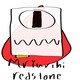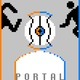# Lists

### Overview

You can make lists in Desmos using square brackets like this:You can create lists with evenly spaced elements. [1,...,10] is a list of the integers between 1 and 10. [1,3,...,11] is a list of the odd integers between 1 and 11. Table columns are available as lists in the rest of the system.

You can use lists anywhere in expressions that you would use a number.### More Resources

2 out of 6 found this helpful
Have more questions? Submit a request

•Abotting213

What is the maximum length of a list? May I have 16,000 elements in it?

•Kashi Yamagato

Can I access an item of a specific index? Say I have a list (or a table) and I want the last item in the list. Is there a way to do this?

•Team Desmos

Kashi - you can find a certain number in a list like x1 will give the 3rd number in the x1 list.

•Aran Glancy

Can you concatenate lists? For example, if I have a=[1,2,3,4] and b=[5,4,3,2,1] and I want to get c=[1,2,3,4,5,4,3,2,1] can I do something like c=a+b?

•Team Desmos

Aran - not currently, but it's on our list!

•MrYurihi redstone

Can you get multiple entries from an list, like getting entries 2 to 6, but in one thing? If not, how could you get Quartile 1 and Quartile 3 from any given list?

Edited by MrYurihi redstone
•Kristin

You can try something like this to reference certain elements in a list: https://www.desmos.com/calculator/zcyahozrpb

Edited by Kristin
•Bryan Garcia

I made two original equations to go from binary to decimal and decimal to binary. The binary to decimal uses the lists feature in desmos. I was wondering if there was any way that I could make a number into a list so that I could just type in the number in a function I guess and use that list instead of putting a comma after every single digit in a 20 digits long binary string.

•Serge Ballif

Bryan Garcia, The trick is to use an intermediary list. I made an example that will convert a decimal whole number to a list of digits. You can modify it as needed. https://www.desmos.com/calculator/7dsepfo2pe

•Serge Ballif

MrYurihi redstoneYou can use the quantile function in the stats menu. To get Q1 and Q3 of a list A you would use the commands quantile(A,.25) and quantile(A,.75). You can even use the quantile function to sort a list. The worksheet https://www.desmos.com/calculator/rfgwycggun shows an example of a sorted list along with a method to concatenate lists or to get a sublist,

•Bombergere23

Aran Glancy,

see here for concatenating lists

•Joe Quinn

I have an equation with 2 parameters (say A and B) in it, and I want to show all the graphs with all combinations of A and B at integers from 1 to 10. If I put A=[1,...,10] and then B=[1,...,10], I only get the graphs for A ranging from 1 to 10 with B fixed at 1. How do I show combinations of choices for the two parameters?

•Serge Ballif

Joe. There are currently no methods for iterating over two lists as you requested. Instead, you could make two lists of length 100 to cover all the ordered pairs. The worksheet https://www.desmos.com/calculator/cg2i9poeub has an example showing how that might be done.

•Joe Quinn

•Bakerjr2159

How do i find quartiles on desmos?

•Serge Ballif

Bakerjr2159 To get quartiles define a list "L=[1,2,3,4,5]" (or table of values with header "L") and enter quantile(L,0.25) to get the first quartile.

•Benjamin Korpella

Abotting213 - No, you can have up to 10000 entries.BTY: if you try to make a list like this, with more than 10000 entries, desmos won't do anything until you reload.

Edited by Benjamin Korpella
•Maxim Tiburziano

Can I set lists to be unbounded between them? If I create a function with two variables and I set the variable A as [0,1,...,9] and B as [0,1,...,9] automatically the result is the combination of the first objects, second objects, third objects and so on.... but I would like the whole possible combination between them Is it possile?

•Serge Ballif

Maxim, there is no way to nest lists, unfortunately. One possible way to get all of the ordered pairs (0,0) through (9,9) is to make use of another list like this link: https://www.desmos.com/calculator/odm1auohqk

•Joe Boccuzzi

Is there a way to find the sum of a list, like [0,1,2,3,4,5] would sum to 15?

•Serge Ballif

total([0,1,2,3,4,5]) will give you the sum of the list.

•Curtis Dyer

Kashi Yamagato: if you want the last element in a list:

L = [0,1,5,9]

S = length(L)

L[S] gives "9", L[S-1] gives "5", and so on.

•dor barlev

Is there any way to reverse a list? Everything I try only sums a list's elements instead of reversing it.

•Serge Ballif

The list reversal question is a good one. I can't think of any satisfying way to do it. You could create an intermediary list and use summation like at https://www.desmos.com/calculator/yhrhf93c6x but it's not particularly pleasing.

•Ryan Delagarza

Can I apply a rule to a list for easy edits of equations?

Ex. X=[1,2,3,4] & Y=2[X]

•Appunosolutions

Hi, I want only one expression. please check the screenshot. please guide me .how can i limit expressions not an expression character. i used desmos api•dor barlev

Ryan Delagarza you can do this:

X = [...]

Y = 2X

This will make Y have a copy of X, with all values multiplied by 2

•Mason Pysell

I am trying to make a formula for a list that uses itself to define the next value, like a recursive formula. For example:

a = [t, 2t+a, 3t+a, 4t+a, ... nt+a[n-1]]

When I attempt something like this in Desmos, I get the following error message:

"Cannot index a number with a number."

Is this error caused by something in my example, or would I need to provide my specific formula?

If it is, how would I get around it?

Thanks,

Mpprogram6771

•Serge Ballif

Mason,

Unfortunately, Desmos doesn't support recursion yet.

•